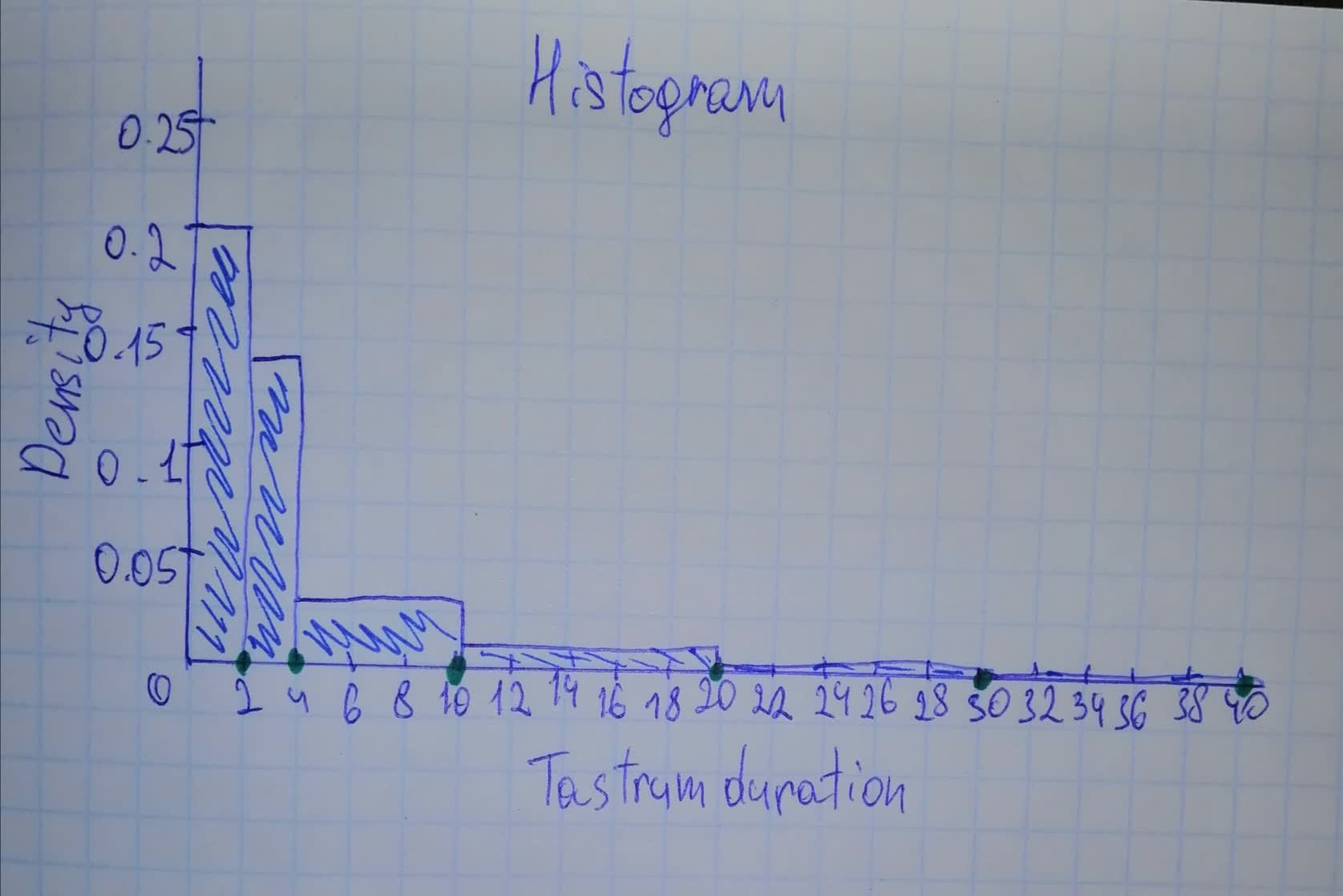# The article “Statistical Modeling of the Time Course of Tantrum Anger” (Annals ojack89515lg 2021-11-18 Answered

The article “Statistical Modeling of the Time Course of Tantrum Anger” (Annals of Applied Stats, 2009: 1013–1034) discussed how anger intensity in children’s tantrums could be related to tantrum duration as well as behavioral indicators such as shouting, stamping, and pushing or pulling. The following frequency distribution was given (and also the cor responding histogram):
$$\begin{array}{|c|c|}\hline 0-<2: & 136 \\ \hline 2-<4: & 92 \\ \hline 4-<11: & 71 \\ \hline 11-<20: & 26 \\ \hline 20-<30: & 7 \\ \hline 30-<40: & 3 \\ \hline \end{array}$$
Draw the histogram and then comment on any interesting features.

You can still ask an expert for help

• Questions are typically answered in as fast as 30 minutes

Solve your problem for the price of one coffee

• Math expert for every subject
• Pay only if we can solve itHarr1957

Step 1
As we can see, the class intervals have unequal lenght, which means that we need to use hisrogram with unequal ckass widths.
Constructing a Histogram for continuous data: Unequal Class Widths:
After determining frequencies and relative frequencies, calculate teh height of each rectangle using the formula
$7\text{rectangle height}=\frac{\text{relative frequency of the class}}{\text{class width}}$
The resulting rectangle height are usually called densities, and the vertical scale is the density scale. This prescription will also work when class widths are equal.
Step 2
Look at the following table:
$\begin{array}{|ccccc|}\hline \text{Interval}& \text{Frequency}& \text{Class Width}& \text{Relative Frequency}& \text{Density}\\ 0-<2:& 136& 2& 0.41& 0.20298\\ 2-<4:& 92& 2& 0.27& 0.13731\\ 4-<11:& 71& 7& 0.21& 0.03028\\ 11-<20:& 26& 9& 0.08& 0.00862\\ 20-<30:& 7& 10& 0.02& 0.00209\\ 30-<40:& 3& 10& 0.01& 0.00089\\ \hline\end{array}$
In the table, intervals and frequencies were given in the exercise.
Class width is difference between upper bound and lower bound.
Relative Frequency is Frequency dividedd by total (sum) of all frequencies which is $335\left(136+92+71+26+7+3\right)$, for example $\frac{136}{335}=0.41$
We get the density column by dividing the Relative Frequency with the Class Width $\left(\frac{0.41}{2}\approx 0.20298\right)$.
Now we have everything we need to create a histogram.
Step 3Step 4
The histogram is positively skewed (more data points on the left side). It is not clear from the histogram to see which value would be representiative (typical) value. The histogram is unimodal (it has only one peak-hump). We can notice that the most tantrums are between 0 and 11 (around $90\mathrm{%}$)
There are only small amount of tantrums that last more than 30 minutes (we can not even see them clearly on the histogram).

###### Not exactly what you’re looking for?Mespirst
Step 1
$\begin{array}{|cc|}\hline n& Frequency\\ 0-2& 136\\ 2-4& 92\\ 4-11& 71\\ 11-20& 26\\ 20-30& 7\\ 30-40& 3\\ \hline\end{array}$